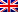# Simulated annealing

Contenus

## Simulated annealing

Annealing is a metallurgical method making it possible to obtain crystallized solids while avoiding the glass state. Once the metal has melted, the temperature is gradually lowered until it reaches a solid state. To remove all defects, the metal is heated and then cooled, in decreasing temperature steps, until a stable state is reached.

This decreasing step system gave birth to the Metropolis algorithm of 1953 and then to the annealing simulated by IBM in 1983.

## Presentation of the algorithm

The simulated annealing algorithm, or simulated annealing for English speakers, is therefore the algorithmic adaptation of the annealing process.

The goal is to achieve a stable state of the objective function - a local or global optimum - from a random solution. This state is reached in stages, where the acceptance of a mutation is made according to the "temperature" of the stage.

The simulated annealing algorithm successively generates configurations from an initial solution R0 and an initial temperature T0 decreasing with each iteration, until a stable configuration is obtained. The probability of accepting a new configuration is (Metropolis rule):

• 1 if the configuration improves the objective function;
• exp (diff (E) / T) with diff (E) the energy difference and T the temperature; the energy difference is an internal function, depending on the objective function.

## Sequence of the algorithm

The annealing algorithm is as follows:

1. Take an initial solution R = R0 and an initial temperature T = T0. The initial state is as for the exact methods, obtained by a heuristic (descent or greedy).
2. Generate a random solution R(i + 1) in the neighborhood of the current solution:
1. compare R(i + 1) with Ri according to the Metropolis rule
2. repeat until a stable solution is found (or after a certain number of iterations)
3. Decrease the temperature T to a threshold temperature Tmin, or have a stable solution.
``` R0
T0
as long as T> Tmin and E> emax
R(i + 1) = neighbor (Ri)
if E (R(i + 1)) <E (Ri) Where random () <P (diff (E) / T) so
accept R(i + 1)
update T
return Rnot```Since T is large at the start, many solutions degrading the current solution can be chosen. This makes it possible to escape from a local optimum. The following figure shows the value of the objective function as a function of the parameter vector X. The temperature therefore makes it possible to “skip” less “good” areas to leave a “valley”. The balls and signals in the following figure show up to what height the balls can "jump", that is to say the tolerance of negative variation of the objective function in obtaining a new solution.If the temperature is low, it is more difficult to escape from a local optimum:FRFRENRUES